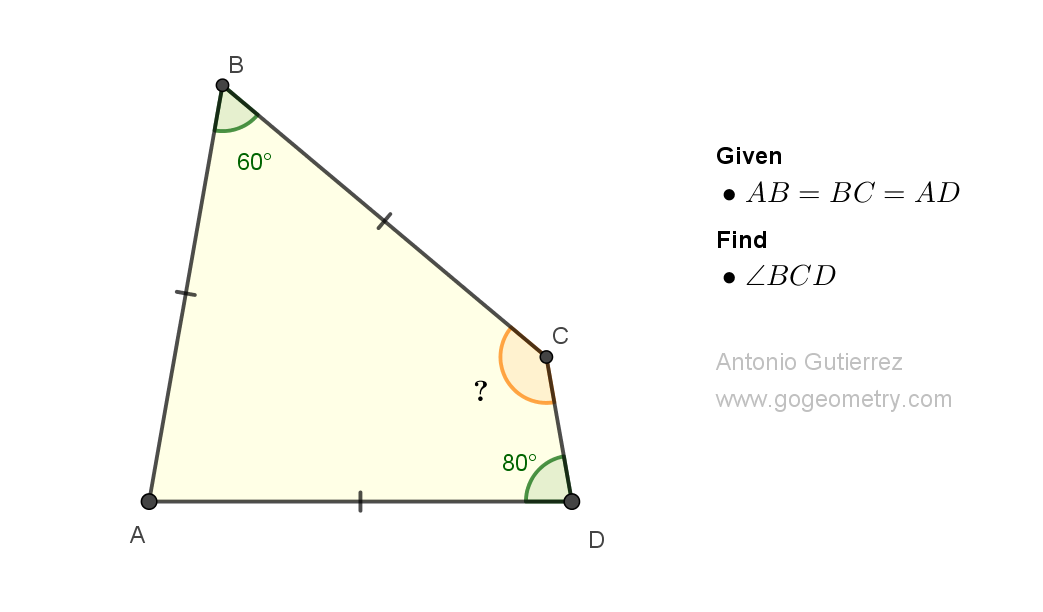# Geometry Problem 1508: Understanding Triangle Geometry: Isosceles, Equilateral, Quadrilateral, Angular. Difficulty Level: High School.

In the figure shown, with angle B = 60 degrees, angle D = 80 degrees, and AB = BC = AD, calculate the measure of angle BCD.## Definitions and Suggestions

• A triangle is a polygon that has three sides, three vertices, and three angles. Triangles are some of the most basic shapes in geometry and can be found in many different forms.
• An angle is a figure formed by two rays, or half-lines, that have a common endpoint and extend in different directions.
• An isosceles triangle is a type of triangle that has two sides of equal length and two angles opposite those sides of equal measure.
• An equilateral triangle is a type of triangle in which all three sides are of equal length, and all three angles are equal to 60 degrees.
• A quadrilateral is a four-sided polygon, which is a two-dimensional geometric figure with straight sides.
• Suggestions:
The sum of the angles in a triangle is always 180 degrees, which is known as the Triangle Sum Theorem.
• The sum of the angles in a quadrilateral is always 360 degrees.